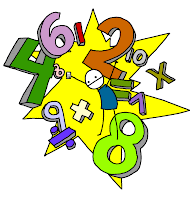"Tiap-tiap diri akan merasai mati. Sesungguhnya akan disempurnakan pahala kamu pada hari kiamat. Sesiapa yang TERSELAMAT DARI NERAKA dan dimasukkan ke dalam syurga, sesungguhnya BERJAYA lah dia, dan ingatlah bahawa kehidupan di dunia ini hanyalah kesenangan yang memperdaya." (Ali Imran : 185)

### Sayyidina Ali (r.a.) and some maths problems

*Sayyidina Ali (rodhiallahu 'anhu) while he was a Khalifa was known for his mathematical genius. One Day a person came to him, thinking that since Ali thinks he is too smart, I'll ask him such a tough question that he won't be able to answer it and I'll have the chance to embarrass him in front of all the Arabs.

He asked "Ali, tell me a number, that if we divide it by any number from 1-10 the answer will always come in the form of a whole number and not as a fraction." Ali Looked back at him and said, "Take the number of days in a year and multiply it with the number of days in a week and you will have your answer."

The person got astonished but as he was a Mushrik he still didn't believe Ali. He calculated the answer Ali gave him. To his amazement he came across the following results:

The number of Days in a Year = 360 (in Arabic Calendar)
The Number of Days in a Week = 7
The product of the two numbers = 2520

Now...

2520 ÷ 1 = 2520
2520 ÷ 2 = 1260
2520 ÷ 3 = 840
2520 ÷ 4 = 630
2520 ÷ 5 = 504
2520 ÷ 6 = 420
2520 ÷ 7 = 360
2520 ÷ 8 = 315
2520 ÷ 9 = 280
2520 ÷ 10= 252

In another occasion...

A person was about to die, and before dying he wrote his Will which went as follows...

"I have 17 Camels, and I have three sons. Divide my Camels in such a way, that My eldest son gets half of them, the second one gets 1/3rd of the total and my youngest son gets 1/9th of the total number of Camels"

After his death when the relatives read his will they got extremely perplexed and said to each other that how can we divide 17 camels like this.

So after a long hard thought they decided that there was only one man in Arabia who could help them: Ali Ibne Abi Taalib.

So they all came to the door of Ali and put forward their problem.

Ali said, "Ok. I will divide the camels as per the man's will. I will lend one of my camels to the total which makes it 18 (17+1), now lets divide as per his will"

The Eldest gets 1/2 of 18 = 9
The second one gets 1/3 of 18 = 6
and The Youngest gets 1/9 of 18 = 2
Now the total number of camels = 17

Then Ali said, "Now I will take my Camel back"

*